Function Repository Resource:

# CombinatorConvert

Convert between different combinator formats

Contributed by: Mano Namuduri and Jan Mangaldan
 ResourceFunction["CombinatorConvert"][cmb,format] converts a combinator expression cmb to the specified format. ResourceFunction["CombinatorConvert"][transform] interconverts a combinator transformation rule transform between the RuleDelayed and Function formats. ResourceFunction["CombinatorConvert"][transform,name] interconverts a combinator transformation rule transform using the symbol name to designate the combinator on the left-hand side when converting to RuleDelayed.

## Details

The following settings for format are supported:
 "Application" combinator represented in terms of Application,S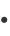(K(SI))(S(KK)I)xy "List" combinator represented as a list, {{{{S, {K, {S,I}}}, {{S, {K,K}}, I}}, x}, y} "Nested" combinator in nested bracket format, S[K[S[I]]][S[K[K]][I]][x][y] "String" combinator represented as a left-associative string, "S(K(SI))(S(KK)I)xy"
Accordingly, the argument cmb can be given in any of the above formats.
If transform is given as a RuleDelayed (lhsrhs), then ResourceFunction["CombinatorConvert"] will return a pure Function. If transform is given as a Function (arg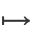body), then ResourceFunction["CombinatorConvert"] will return a RuleDelayed.
The argument name, if specified, should be given as a Symbol (default is λ).

## Examples

### Basic Examples (5)

Convert a combinator expression in application format to each of the possible formats:

 In:=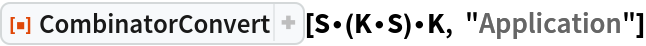Out=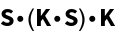In:=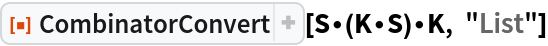Out=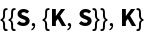In:=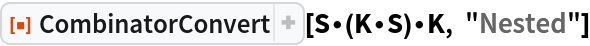Out=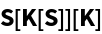In:=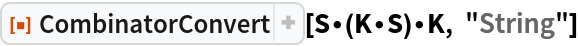Out=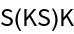Convert a combinator expression in nested bracket format to each of the possible formats:

 In:=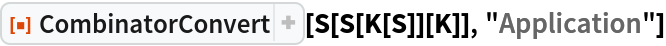Out=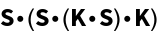In:=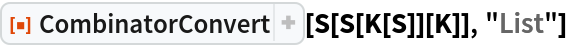Out=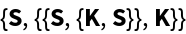In:=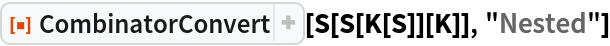Out=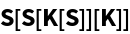In:=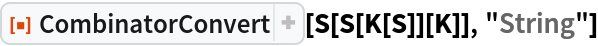Out=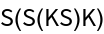Convert a combinator expression in list format to each of the possible formats:

 In:=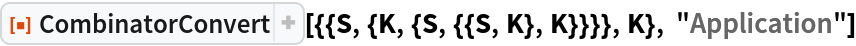Out=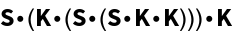In:=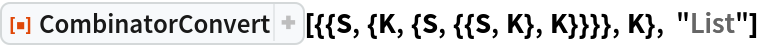Out=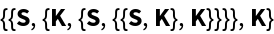In:=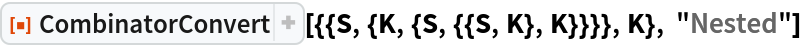Out=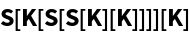In:=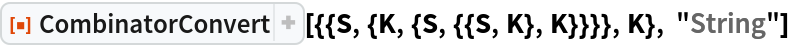Out=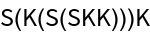Convert a combinator expression in list format to each of the possible formats (note that the conversion from string format only allows single-character symbols, so combinator glyphs would need to be inserted retroactively):

 In:=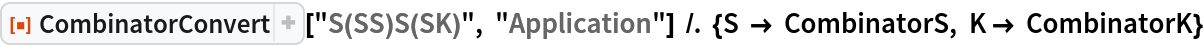Out=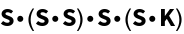In:=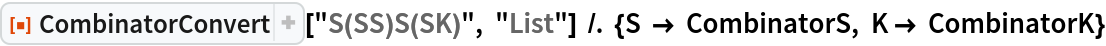Out=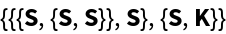In:=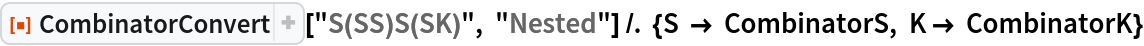Out=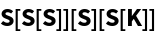In:=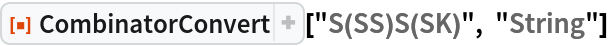Out=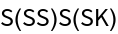Convert between the RuleDelayed and Function formats of the CombinatorB transformation:

 In:=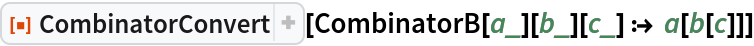Out=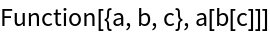In:=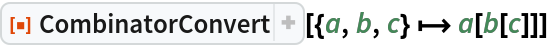Out=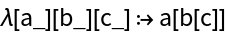When converting from Function to RuleDelayed, a second argument must be supplied for the correct symbol to appear on the left-hand side:

 In:=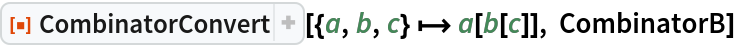Out=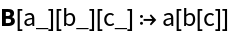### Possible Issues (3)

Input strings containing any symbol other than letters, digits or parentheses are currently not supported:

 In:=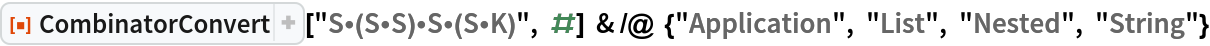Out=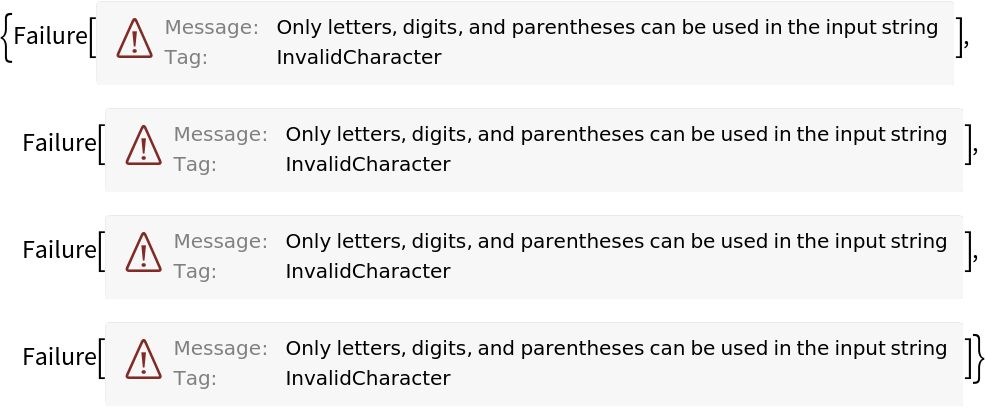Input strings must be in left-associative format:

 In:=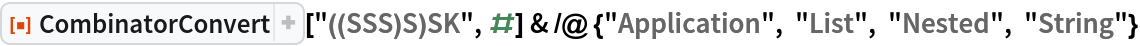Out=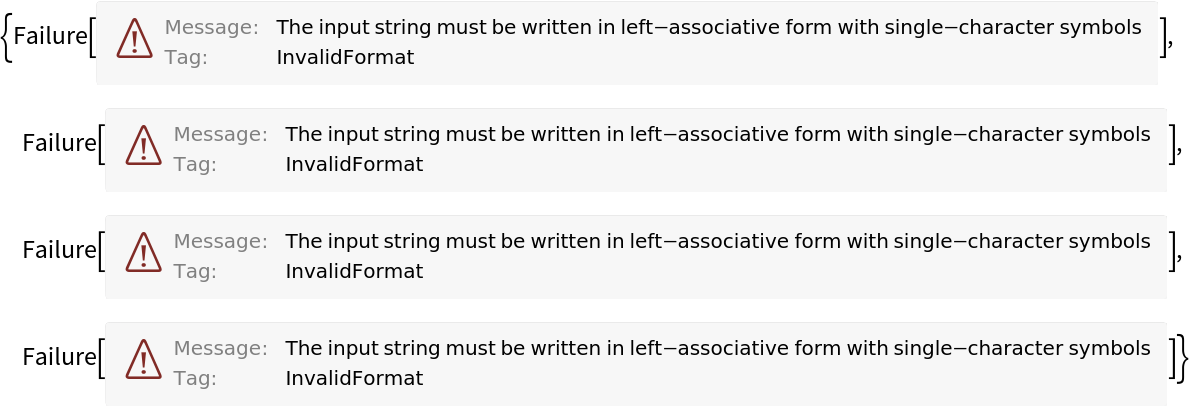A combinator transformation must be a RuleDelayed or Function object:

 In:=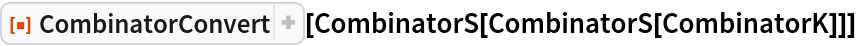Out=## Version History

• 1.0.0 – 10 March 2021